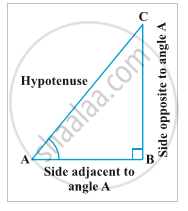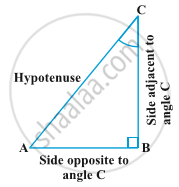Karnataka Secondary Education Examination BoardKarnataka SSLC

# Trigonometric Ratios and Its Reciprocal

## Definition

Trignometric ratios are ratios of two sides of a right triangle and a related angle.

## Notes

A]Trignometric ratios of an acute angle of a right triangle-Let take A as θ

AC is a hypotenuse. Hypotenuse is the longest side in a right angled triangle and it is opposite of the right angle.

Now, here θ will decide the base and perpendicular in a right angled triangle.

The side on which θ lies is known as base, and the side opposite to θ is known as perpendicular. In the above figure AB is the base and BC is the perpendicular.

There exist six trignometric ratios, sin, cos, tan, cot, sec and cosec, and they are as follows,

1)sinθ= "Perpendicular"/"hypotenuse" = "BC"/"AC"

2)cosθ= "base"/ "hypotenuse"= "AB"/"AC"

3)tanθ= "perpendicular"/"base"= "BC"/"AB"

4)cotθ= "base"/ "perpendicular"= "AB"/"BC"

5)secθ= "hypotenuse"/ "base"= "AC"/"AB"

6)cosecθ= "hypotenuse"/ "perpendicular"= "AC"/"BC"

Similarly, if we take,Here, Let take C as θ

AC is the hypotenuse, BC is the base and AB is the perpendicular.

The ratios will be,

1)sinθ= "AB"/"AC"

2)cosθ= "BC"/"AC"

3)tanθ= "AB"/"BC"

4)cotθ= "BC"/"AB"

5)secθ= "AC"/"BC"

6)cosecθ= "AC"/"AB"

B] Reciprocal Relation-

1) cosecθ= 1/sin theta

2) secθ= 1/ cos theta

3) cotθ= 1/tan theta

4) sinθ= 1/(cosec theta)

5) cosθ= 1/sec theta

6) tanθ= 1/cot theta

C] Power of Trigonometric ratios-

1) (sin theta)^2= sin^2 theta

2) sin^2 theta= (sin theta)^2

3) (cos theta)^3= cos^3 theta

If you would like to contribute notes or other learning material, please submit them using the button below.